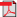# Search Results

1. J. Nousiainen, J. Virtamo and P. Lassila, Maximum Weight Independent Sets in an Infinite Plane, in 21st International Teletraffic Congress (ITC 21), pp. 1-8, 2009, Paris, France, (received the Best Student Paper Award)(bib)
Abstract: We study the maximum weight independent sets of links between nodes distributed as a spatial Poisson process in an infinite plane. Three different definitions of the weight of a link are considered, leading to slight variations of what is essentially a spatial reuse problem in wireless multihop networks. A simple Boolean interference model is assumed with the interference radius equaling the transmission radius. We study both the case where the transmission radius is fixed and the case where it can be reduced (by power control) so as to just reach the destination to minimize the interference. For the case of a fixed transmission radius, we give asymptotic results for the low density regime and present a rudimentary analysis for the high density asymptotics. The main contribution of this paper is in the numerical results for the maximum weight for the considered infinite networks and in thus establishing some previously unknown parameters of stochastic geometry. For instance, we find that in the unweighted case, just counting the number of independent links, the maximum possible packing is 0.322 links per node attained with the mean neighborhood size of 2.73. The results are obtained by the Moving Window Algorithm that is able to find the maximum weight independent set of a network realization in a strip of limited height but unlimited length. By studying the results as the function of the height of the strip, we are able to extrapolate to the infinite plane.